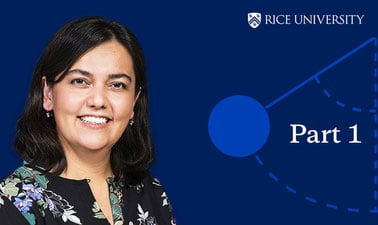Ir al contenido principal

# Ordinary Differential Equations and Linear Algebra - Part 1

Study of ordinary differential equations (e.g., solutions to separable and linear first-order equations and to higher-order linear equations with constant coefficients, systems of linear differential equations, the properties of solutions to differential equations) and linear algebra (e.g., vector spaces and solutions to algebraic linear equations, dimension, eigenvalues, and eigenvectors of a matrix), as well as the application of linear algebra to first-order systems of differential equations and the qualitative theory of nonlinear systems and phase portraits.

...